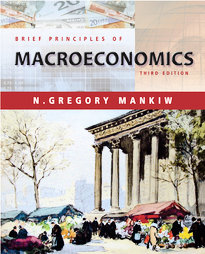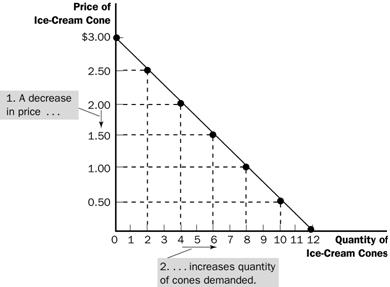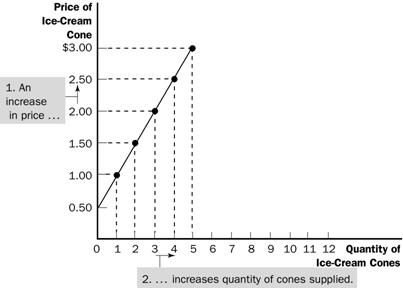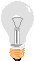CHAPTER 4 SUPPLY AND DEMAND BRIEF PRINCIPLES OF MACROECONOMICS

LEARNING OBJECTIVES:

By the end of this chapter, you should understand:

Ø       what a competitive market is.

Ø       what determines the demand for a good in a competitive market.

Ø       what determines the supply of a good in a competitive market.

Ø       how supply and demand together set the price of a good and the quantity sold.

Ø       the key role of prices in allocating scarce resources in market economies.

KEY POINTS:

1.       Economists use the model of supply and demand to analyze competitive markets. In a competitive market, there are many buyers and sellers, each of whom has little or no influence on the market price.

2.       The demand curve shows how the quantity of a good demanded depends on the price. According to the law of demand, as the price of a good falls, the quantity demanded rises. Therefore, the demand curve slopes downward.

3.       In addition to price, other determinants of how much consumers want to buy include income, the prices of substitutes and complements, tastes, expectations, and the number of buyers. If one of these factors changes, the demand curve shifts.

4.       The supply curve shows how the quantity of a good supplied depends on the price. According to the law of supply, as the price of a good rises, the quantity supplied rises. Therefore, the supply curve slopes upward.

5.       In addition to price, other determinants of how much producers want to sell include input prices, technology, expectations, and the number of sellers. If one of these factors changes, the supply curve shifts.

6.       The intersection of the supply and demand curves determines the market equilibrium. At the equilibrium price, the quantity demanded equals the quantity supplied.

7.       The behavior of buyers and sellers naturally drives markets toward their equilibrium. When the market price is above the equilibrium price, there is a surplus of the good, which causes the market price to fall. When the market price is below the equilibrium price, there is a shortage, which causes the market price to risE

8.       To analyze how any event influences a market, we use the supply-and-demand diagram to examine how the event affects equilibrium price and quantity. To do this we follow three steps. First, we decide whether the event shifts the supply curve or the demand curve (or both). Second, we decide which direction the curve shifts. Third, we compare the new equilibrium with the initial equilibrium.

9.       In market economies, prices are the signals that guide economic decisions and thereby allocate scarce resources. For every good in the economy, the price ensures that supply and demand are in balance. The equilibrium price then determines how much of the good buyers choose to purchase and how much sellers choose to produce.

## CHAPTER OUTLINE:

I.          Markets and Competition

A.         What Is a Market?

1.         Definition of market: a group of buyers and sellers of a particular good or service.

2.         Markets can take many forms and may be organized (agricultural commodities) or less organized (ice cream).

B.         What Is Competition?

1.         Definition of competitive market: a market in which there are so many buyers and so many sellers that each has a negligible impact on the market price.

2.         Each buyer knows that there are several sellers from which to choose. Sellers know that each buyer purchases only a small amount of the total amount sold.

C.         In this chapter, we will assume that markets are perfectly competitive.

1.         Characteristics of a perfectly competitive market:

a.         The goods being offered for sale are exactly the same.

b.         The buyers and sellers are so numerous that no single buyer or seller has any influence over the market price.

2.         Because buyers and sellers must accept the market price as given, they are often called "price takers."

3.         Not all goods are sold in a perfectly competitive market.

a.         A market with only one seller is called a monopoly market.

b.         Some markets fall between perfect competition and monopoly.

D.         We will start by studying perfect competition.

1.         Perfectly competitive markets are the easiest to analyze because buyers and sellers take the price as a given.

2.         Because some degree of competition is present in most markets, many of the lessons that we learn by studying supply and demand under perfect competition apply in more complicated markets.

II.         Demand

A.         The Demand Curve: The Relationship between Price and Quantity Demanded

1.         Definition of quantity demanded: the amount of a good that buyers are willing and able to purchase.

2.         One important determinant of quantity demanded is the price of the product.

a.         Quantity demanded is negatively related to price. This implies that the demand curve is downward sloping.

b.         Definition of law of demand: the claim that, other things being equal, the quantity demanded of a good falls when the price of the good rises.

3.         Definition of demand schedule: a table that shows the relationship between the price of a good and the quantity demanded.

 Price of Ice Cream Cone Quantity of Cones Demanded \$0.00 12 \$0.50 10 \$1.00 8 \$1.50 6 \$2.00 4 \$2.50 2 \$3.00 0

4.         Definition of demand curve: a graph of the relationship between the price of a good and the quantity demanded.

a.         Price is generally drawn on the vertical axis.

b.         Quantity demanded is represented on the horizontal axis.B.         Market Demand versus Individual Demand

1.         The market demand is the sum of all of the individual demands for a particular good or service.

2.         The demand curves are summed horizontally—meaning that the quantities demanded are added up for each level of price.

3.         The market demand curve shows how the total quantity demanded of a good varies with the price of the good, holding constant all other factors that affect how much consumers want to buy.

C.         Shifts in the Demand Curve

1.         The demand curve shows how much consumers want to buy at any price, holding constant the many other factors that influence buying decisions.

2.         If any of these other factors change, the demand curve will shift.

a.         An increase in demand is represented by a shift of the demand curve to the right.

b.         A decrease in demand is represented by a shift of the demand curve to the left.

3.         Income

a.         The relationship between income and quantity demanded depends on what type of good the product is.

b.         Definition of normal good: a good for which, other things being  equal, an increase in income leads to an increase in demand.

c.          Definition of inferior good: a good for which, other things being equal, an increase in income leads to a decrease in demand.

4.         Prices of Related Goods

a.         Definition of substitutes: two goods for which an increase in the price of one good leads to an increase in the demand for the other.

b.         Definition of complements: two goods for which an increase in the price of one good leads to a decrease in the demand for the other.

5.         Tastes

6.         Expectations

a.         Future Income

b.         Future Prices

D.         Case Study: Two Ways to Reduce the Quantity of Smoking Demanded

1.         Public service announcements, mandatory health warnings on cigarette packages, and the prohibition of cigarette advertising on television are policies designed to reduce the demand for cigarettes (and shift the demand curve to the left).

2.         Raising the price of cigarettes (through tobacco taxes) lowers the quantity of cigarettes demanded.

a.         The demand curve does not shift in this case, however.

b.         An increase in the price of cigarettes can be shown by a movement along the original demand curve.

3.         Studies have shown that a 10% increase in the price of cigarettes causes a 4% reduction in the quantity of cigarettes demanded. For teens, a 10% increase in price leads to a 12% drop in quantity demanded.

4.         Studies have also shown that a decrease in the price of cigarettes is associated with greater use of marijuana. Thus, it appears that tobacco and marijuana are complements.

III.       Supply

A.         The Supply Curve: The Relationship between Price and Quantity Supplied

1.         Definition of quantity supplied: the amount of a good that sellers are willing and able to sell.

a.         Quantity supplied is positively related to price. This implies that the supply curve will be upward sloping.

b.         Definition of law of supply: the claim that, other things being  equal, the quantity supplied of a good rises when the price of the good rises.

2.         Definition of supply schedule: a table that shows the relationship between the price of a good and the quantity supplied.

3.         Definition of supply curve: a graph of the relationship between the price of a good and the quantity supplied.

 Price of Ice Cream Cone Quantity of Cones Supplied \$0.00 0 \$0.50 0 \$1.00 1 \$1.50 2 \$2.00 3 \$2.50 4 \$3.00 5B.         Market Supply versus Individual Supply

1.         The market supply curve can be found by summing individual supply curves.

2.         Individual supply curves are summed horizontally at every price.

3.         The market supply curve shows how the total quantity supplied varies as the price of the good varies.

C.         Shifts in the Supply Curve

1.         The supply curve shows how much producers offer for sale at any given price, holding constant all other factors that may influence producers’ decisions about how much to sell.

2.         When any of these other factors change, the supply curve will shift.

a.         An increase in supply is represented by a shift of the supply curve to the right.

b.         A decrease in supply is represented by a shift of the supply curve to the left.

3.         Input Prices

4.         Technology

5.         Expectations

6.         Number of Sellers

IV.        Supply and Demand Together

A.         Equilibrium

1.         The point where the supply and demand curves intersect is called the market’s equilibrium.

2.         Definition of equilibrium: a situation in which the market price has reached the level at which quantity supplied equals quantity demanded.

3.         Definition of equilibrium price: the price that balances quantity supplied and quantity demanded.

4.         The equilibrium price is often called the "market-clearing" price because both buyers and sellers are satisfied at this price.5.         Definition of equilibrium quantity: the quantity supplied and the quantity demanded at the equilibrium price.

6.         If the actual market price is higher than the equilibrium price, there will be a surplus of the good.

a.         Definition of surplus: a situation in which quantity supplied is greater than quantity demanded.

b.                   To eliminate the surplus, producers will lower the price until the market reaches equilibrium.

7.         If the actual price is lower than the equilibrium price, there will be a shortage of the good.

a.         Definition of shortage: a situation in which quantity demanded is greater than quantity supplied.

b.                   Sellers will respond to the shortage by raising the price of the good until the market reaches equilibrium.8.         Definition of the law of supply and demand: the claim that the price of any good adjusts to bring the supply and demand for that good into balance.

B.         Three Steps to Analyzing Changes in Equilibrium

1.         Decide whether the event shifts the supply or demand curve (or perhaps both).

2.         Determine the direction in which the curve shifts.

3.         Use the supply-and-demand diagram to see how the shift changes the equilibrium price and quantity.

C.         Example: A Change in Demand — the effect of hot weather on the market for ice cream.

D.         Shifts in Curves versus Movements along Curves

1.         A shift in the demand curve is called a "change in demand." A shift in the supply curve is called a "change in supply."You should not think about the curves shifting “up” and “down” but rather think about the curves shifting “right” and “left” (or “out” and “in”). Point out that an increase in demand (or supply) is an increase in the quantity demanded (supplied) at every price. Thus, it is quantity that is getting larger. Review the same principle with a decrease in demand (or supply).

2.         A movement along a fixed demand curve is called a "change in quantity demanded." A movement along a fixed supply curve is called a "change in quantity supplied."

E.                   Example: A Change in Supply — the effect of a hurricane that destroys part of the sugar-cane crop and drives up the price of sugar.

F.         In the News: Political Unrest Shifts the Supply Curve

1.         Newspaper articles about specific industries can give students practice understanding the things that affect supply and demand.

2.         This is an article from the Boston Herald that describes the effect of political unrest on the market for cocoa.

G.         Example: A Change in Both Supply and Demand—the effect of both hot weather and a hurricane that destroys part of the sugar cane crop.

H.         Summary

1.         When an event shifts the supply or demand curve, we can examine the effects on the equilibrium price and quantity.

2.         Table 4 reports the end results of these shifts in supply and demand.

V.         Conclusion: How Prices Allocate Resources

A.         The model of supply and demand is a powerful tool for analyzing markets.

B.         Supply and demand together determine the prices of the economy’s goods and services.

1.         These prices serve as signals that guide the allocation of scarce resources in the economy.

2.         Prices determine who produces each good and how much of each good is produced.